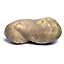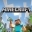# 0.5 is 1/2 and 0.2 is 1/5.Added by a Guest on February 18, 2021 | You Like This |when the math is sus.

unfunnymemelord on February 23, 2021Why would they change math? MATH IS MATH!

B) on February 25, 2021Ok here we go. 1/2=0.5 1/4=0.25 1/8=0.125 1/16=0.0625 1/32=0.03125 5=5^1 25=5^2 125=5^3 625=5^4 3125=5^5 1/5=0.2 1/25=0.04 1/125=0.008 1/625=0.00016 1/3125=0.000032 2=2^1 4=2^2 8=2^3 16=2^4 32=2^5 For starters lol

Ian Ong on May 23, 2021Don't be a potato! Just register or login before commenting.